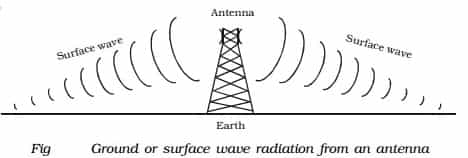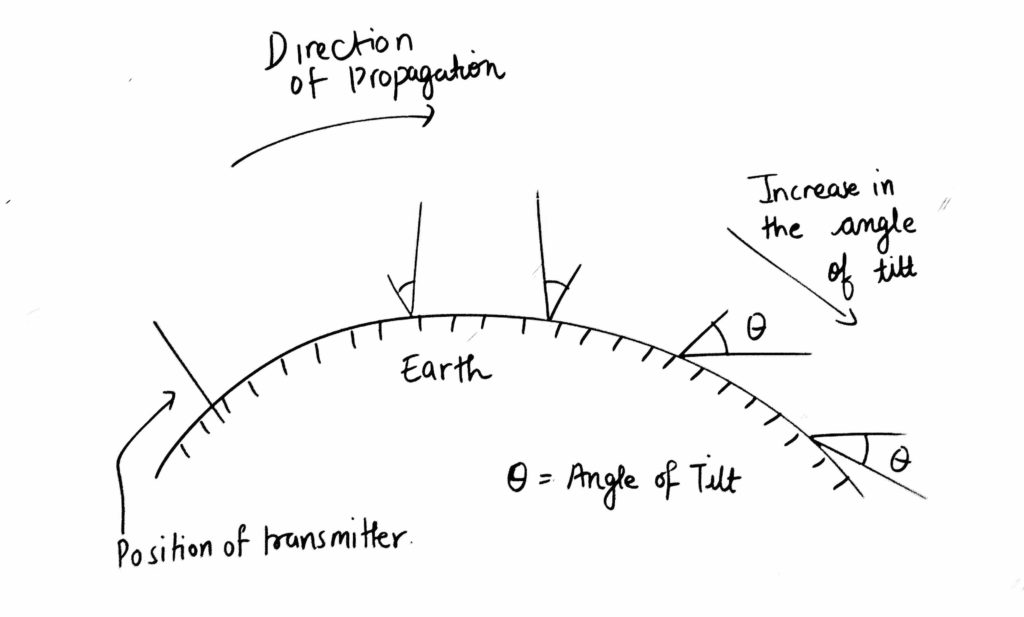# Ground Wave Propagation & Angle of Tilt1. The propagation of electromagnetic waves near the surface of the earth is known as ground wave propagation. It is also known as surface wave propagation.

2. A wave is said to be a ground wave or surface wave when it propagates from the transmitter to receiver by gliding over the surface of the earth and follow the curvature of the earth.3. These waves exist when

• both transmitting and receiving antennas are close to the earth.
• the antennas are vertically polarised.

4. Horizontally polarized antennas are not preferred as the horizontal component of the electric field is contact with the earth is short-circuited by the earth.

5. Ground wave is attenuated as it propagates due to imperfect nature of the earth.

6. Frequency range is 30 KHz to 3MHz. At higher frequencies beyond 3MHz, the earth begins to attenuate the radio signals. It is suitable for VLF, LF and MF communications.

7. The distance of propagation is limited to few hundred kilometres.

8. Ground Wave Field Strength for a flat earth is given by:

E = AEo/d

Where,

E = field strength at a point (V/m)

Eo = field strength of a wave at a unit distance from the Tx Antenna, neglecting earth losses.

A = factor of the ground losses

D = distance of the point from Tx Antenna.

9. Applications:

• Used in marine and navigational aids.
• Used in long distance point to point communication such as ship-to-shore communication.

10. Attenuation of Surface Waves:

• Surface wave induces currents in the ground while travelling over the earth’s surface and thus loses some energy by absorption.
• Due to diffraction, the wavefronts will tilt over the surface. This angle of tilt goes on increasing due to the curvature of the earth surface. So waves lie down and die.

11. The angle of Tilt:

• Wave tilt is defined as the change of orientation of the vertically polarized ground wave at the surface of the earth. This occurs due to diffraction.
• Due to tilt, both horizontal and vertical components of the electric field exists and are not in phase. This tilt changes the originally vertically polarized wave into an elliptically polarized wave.
• As the wave progresses, it tilts over the surface of the earth. This results in power dissipation and ultimately waves lie down and die.### 1 thought on “Ground Wave Propagation & Angle of Tilt”

1.could you please give formulae to calculate wave tilt

This site uses Akismet to reduce spam. Learn how your comment data is processed.

error: